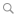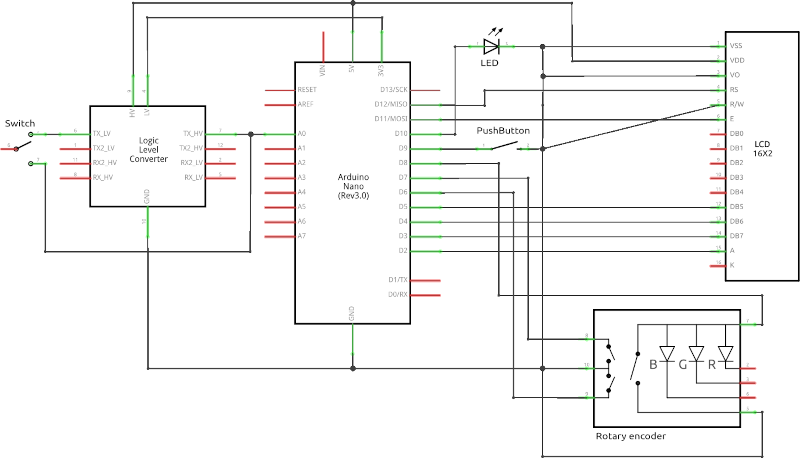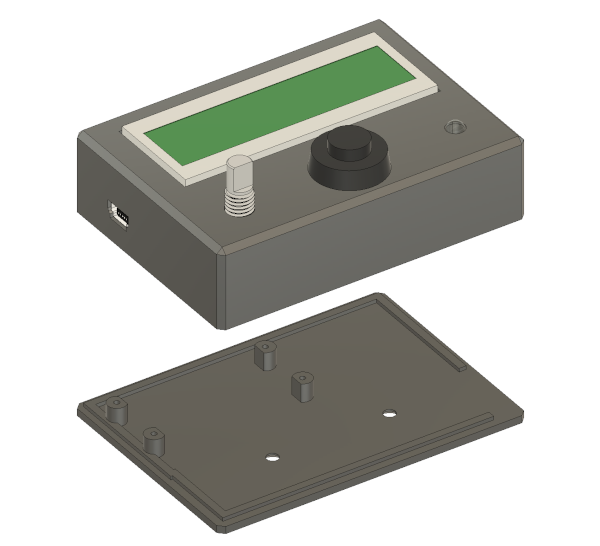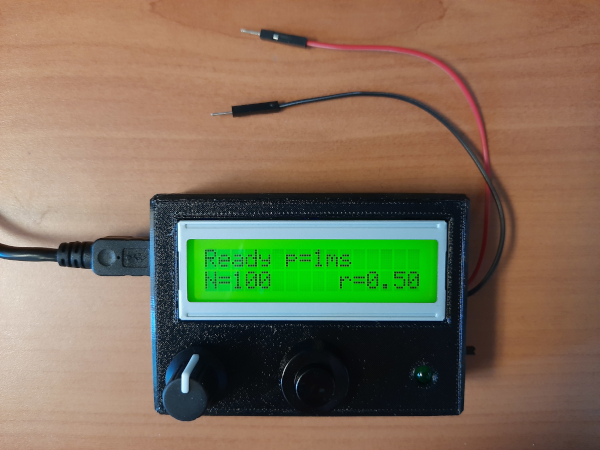BlogA simple digital generator

By Raphaël - August 26th, 2022

0 Comment

In this project I developped a simple yet powerful digital (TTL) generator. Built around an Arduino Nano, the generator fits in a small portable 3d-printed package but it features single step or square-formed periodic signals, manual control of the delivering time or a preset number of periods, and control via the serial connection as well as a LCD with a selectable menu. Last but not least: there is a switch to choose between 3.3V and 5V.

The resulting device reveals to be quite handy and allows me to test components, debug digital circuits or set-up a quick and dirty solution to trig a camera or run a step motor (e.g. with an EasyDriver board).

# Electronics

Let's start with the electronics at play here. Here is the scheme:and the wiring diagram:# Packaging

I designed a special enclosure so that everything can be rassembled in a tiny package and 3D printed it in PETG with a Prusa Mini.

Here is the conception file of the enclosure, in case you'd want to replicate if: BOX.step.3D view of the enclosure. The lid is tightened with two M3 screws into threaded inserts.

# Programming

The code is designed to run on an Arduino Nano rev3.0, and you'll need the LiquidCrystal library. The serial interface is based on my basic Arduino serial server post.

Here is the Arduino sketch:

File: Sketch.ino
/*
* SIMPLE LOGIC GENERATOR
*
*
* Raphaël Candelier, Sorbonne Université, CNRS.
* https://www.labojeanperrin.fr/
*/

// --- Libraries

#include <LiquidCrystal.h>

// --- Definitions

#define SRC_BTN 0
#define SRC_SRL 1
#define RUNNING 1
#define PERIOD 2
#define NUMBER 3
#define RATIO 4
#define PERIOD_1 5
#define PERIOD_10 6
#define PERIOD_100 7
#define PERIOD_1000 8
#define N_0 9
#define N_1 10
#define N_10 11
#define N_100 12
#define RATIO_FULL 13
#define RATIO_HALF 14
#define RATIO_10 15

// Button
const int BT = 9;

// Rotary encoder
const int CLK = 6;
const int DT = 7;
const int SW = 8;

int counter = 0;
int lastStateCLK;
unsigned long lastKB = 0;

// LCD
const int D7 = 2;
const int D6 = 3;
const int D5 = 4;
const int D4 = 5;
const int EN = 11;
const int RS = 12;
LiquidCrystal lcd(RS, EN, D4, D5, D6, D7);

// LED
const int SL = 10;

// OUTPUT
const int OUT = A0;

// DEFAULT VALUES
unsigned long int period = 1000000;   // microseconds
float ratio = 1;
unsigned int N = 0;

// STATES

// MISC
unsigned long trigTime;
int trigSrc;

// --- SETUP ----------------------------------------------------------

void setup() {

// Pins
pinMode(BT, INPUT_PULLUP);
pinMode(CLK,INPUT_PULLUP);
pinMode(DT,INPUT_PULLUP);
pinMode(SW, INPUT_PULLUP);
pinMode(SL, OUTPUT);
pinMode(OUT, OUTPUT);

// Serial Monitor
Serial.begin(115200);
Serial.setTimeout(5);

// Rotary encoder

// --- LCD display

// Definition
lcd.begin(16, 2);
startup_animation();

}

void startup_animation() {

String s0 = "  SIMPLE LOGIC  ";
String s1 = "   GENERATOR    ";
int tw = 75;

lcd.clear();

for (int i=0; i<16; i++) {
lcd.setCursor(i,0);
lcd.print(s0.charAt(i));
if (i<15) { lcd.print(">"); }
lcd.setCursor(i,1);
lcd.print(s1.charAt(i));
if (i<15) { lcd.print(">"); }
delay(tw);
}

delay(1000);
UpdateLCD();
}

void start() {

trigTime = micros();
state = RUNNING;
digitalWrite(OUT, HIGH);
digitalWrite(SL, HIGH);

}

void output() {

// Current time
unsigned long t = micros();

if (N==0) {

if ((t-trigTime) % period <= period*ratio) {
digitalWrite(OUT, HIGH);
} else {
digitalWrite(OUT, LOW);
}

} else {

// Stop condition
if (t >= trigTime + period*N) {
stop();
while (trigSrc==SRC_BTN && digitalRead(BT)==LOW) { delay(1); }
} else if ((t-trigTime) % period <= period*ratio) {
digitalWrite(OUT, HIGH);
} else {
digitalWrite(OUT, LOW);
}

}
}

void stop() {

digitalWrite(OUT, LOW);
digitalWrite(SL, LOW);
UpdateLCD();
delay(200);
}

void UpdateLCD() {

lcd.clear();

switch (state) {

lcd.setCursor(0,0);
lcd.print(period/1000);
lcd.print("ms");

lcd.setCursor(0,1);
lcd.print("N=");
lcd.print(N);

lcd.setCursor(10,1);
lcd.print("r=");
lcd.print(ratio);
break;

case RUNNING:

lcd.setCursor(0,0);
lcd.print(">RUN< p=");
lcd.print(period/1000);
lcd.print("ms");

lcd.setCursor(0,1);
lcd.print("N=");
lcd.print(N);

lcd.setCursor(10,1);
lcd.print("r=");
lcd.print(ratio);
break;

case PERIOD:

lcd.print("Period");
break;

case PERIOD_1:

lcd.print("Set period at");
lcd.setCursor(6,1);
lcd.print("1 ms");
break;

case PERIOD_10:

lcd.print("Set period at");
lcd.setCursor(5,1);
lcd.print("10 ms");
break;

case PERIOD_100:

lcd.print("Set period at");
lcd.setCursor(4,1);
lcd.print("100 ms");
break;

case PERIOD_1000:

lcd.print("Set period at");
lcd.setCursor(3,1);
lcd.print("1000 ms");
break;

case NUMBER:

lcd.print("Number");
break;

case N_0:

lcd.print("Set number at");
lcd.setCursor(5,1);
lcd.print("0");
break;

case N_1:

lcd.print("Set number at");
lcd.setCursor(5,1);
lcd.print("1");
break;

case N_10:

lcd.print("Set number at");
lcd.setCursor(4,1);
lcd.print("10");
break;

case N_100:

lcd.print("Set number at");
lcd.setCursor(3,1);
lcd.print("100");
break;

case RATIO:

lcd.print("Ratio");
break;

case RATIO_FULL:

lcd.print("Set full ratio");
lcd.setCursor(6,1);
lcd.print("1");
break;

case RATIO_HALF:

lcd.print("Set half ratio");
lcd.setCursor(5,1);
lcd.print("0.5");
break;

case RATIO_10:

lcd.print("Set small ratio");
lcd.setCursor(5,1);
lcd.print("0.1");
break;

}
}

// --- MAIN LOOP ------------------------------------------------------

void loop() {

/* === SERIAL INPUT =================================== */

if (Serial.available()) {

input.trim();

// --- Display info
if (input.equals("info")) {

Serial.println("----------------------------");
Serial.println("SLG");
Serial.println("N:" + String(N));
Serial.println("Period: " + String(period/1000) + " ms");
Serial.println("Ratio: " + String(ratio));
Serial.println("----------------------------");

// --- Start
} else if (input.equals("start")) {

start();
trigSrc = SRC_SRL;

// --- Stop
} else if (input.equals("stop")) {

if (trigSrc==SRC_SRL) { stop(); }

// --- N
} else if (input.substring(0,1).equals("N")) {

N = input.substring(2).toInt();
UpdateLCD();

// --- Period
} else if (input.substring(0,6).equals("period")) {

period = input.substring(7).toInt()*1000;
UpdateLCD();

// --- Ratio
} else if (input.substring(0,5).equals("ratio")) {

ratio = input.substring(6).toFloat();
UpdateLCD();

}

}

/* === RUN & STOP ===================================== */

// Manual stop
if (state==RUNNING && trigSrc==SRC_BTN && N==0 && !bt) { stop(); }

if (state==RUNNING) {
output();
return;
}

/* === MECHANICAL INPUT =============================== */

// --- Rotary encoder

int inc = 0;
bool kb = false;

// --- Rotary encoder increment

if (currentStateCLK != lastStateCLK  && currentStateCLK == 1){
if (digitalRead(DT) != currentStateCLK) { inc = -1; }
else { inc = 1; }
}
lastStateCLK = currentStateCLK;

// --- Knob switch

if (millis() > lastKB + 50) {
kb = true;
}
lastKB = millis();
}

// Slight delay to help debounce the increment
delay(1);

/* === STATE MACHINE ================================== */

if (inc || kb || bt) {

switch(state) {

if (kb) {}
if (bt) {
start();
trigSrc = SRC_BTN;
}
if (inc==-1) { state = RATIO; }
if (inc==1) { state = PERIOD; }
break;

case PERIOD:

if (kb) { state = READY; }
if (bt) { state = PERIOD_1; }
if (inc==-1) { state = READY; }
if (inc==1) { state = NUMBER; }
break;

case NUMBER:

if (kb) { state = READY; }
if (bt) { state = N_0; }
if (inc==-1) { state = PERIOD; }
if (inc==1) { state = RATIO; }
break;

case RATIO:

if (kb) { state = READY; }
if (bt) { state = RATIO_FULL; }
if (inc==-1) {state = NUMBER; }
if (inc==1) { state = READY; }
break;

case PERIOD_1:

if (kb) { state = PERIOD; }
if (bt) {
period = 1000;
}
if (inc==-1) { state = PERIOD_1000; }
if (inc==1) { state = PERIOD_10; }
break;

case PERIOD_10:

if (kb) { state = PERIOD; }
if (bt) {
period = 10000;
}
if (inc==-1) { state = PERIOD_1; }
if (inc==1) { state = PERIOD_100; }
break;

case PERIOD_100:

if (kb) { state = PERIOD; }
if (bt) {
period = 100000;
}
if (inc==-1) { state = PERIOD_10; }
if (inc==1) { state = PERIOD_1000; }
break;

case PERIOD_1000:

if (kb) { state = PERIOD; }
if (bt) {
period = 1000000;
}
if (inc==-1) { state = PERIOD_100; }
if (inc==1) { state = PERIOD_1; }
break;

case N_0:

if (kb) { state = NUMBER; }
if (bt) {
N = 0;
}
if (inc==-1) { state = N_100; }
if (inc==1) { state = N_1; }
break;

case N_1:

if (kb) { state = NUMBER; }
if (bt) {
N = 1;
}
if (inc==-1) { state = N_0; }
if (inc==1) { state = N_10; }
break;

case N_10:

if (kb) { state = NUMBER; }
if (bt) {
N = 10;
}
if (inc==-1) { state = N_1; }
if (inc==1) { state = N_100; }
break;

case N_100:

if (kb) { state = NUMBER; }
if (bt) {
N = 100;
}
if (inc==-1) { state = N_10; }
if (inc==1) { state = N_0; }
break;

case RATIO_FULL:

if (kb) { state = RATIO; }
if (bt) {
ratio = 1;
}
if (inc==-1) { state = RATIO_10; }
if (inc==1) { state = RATIO_HALF; }
break;

case RATIO_HALF:

if (kb) { state = RATIO; }
if (bt) {
ratio = 0.5;
}
if (inc==-1) { state = RATIO_FULL; }
if (inc==1) { state = RATIO_10; }
break;

case RATIO_10:

if (kb) { state = RATIO; }
if (bt) {
ratio = 0.1;
}
if (inc==-1) { state = RATIO_HALF; }
if (inc==1) { state = RATIO_FULL; }
break;

}

UpdateLCD();
if (state!=RUNNING) { delay(250); }

}

}

# Usage

When the device is ready (indicated by Ready in the top-left corner of the LCD), output generation can be trigged either by pressing the central button or by sending the start command over the serial channel.

When the menu is explored (via the knob) the device is not ready and no output can be generated; the central button is then used for validation, while the knob button is used for moving back.

There are three parameters that control the output:

• number (Menu) or N (Serial): the number of periods to generate. If N=0, the output is generated until the stop command is sent or the central button is released. Otherwise, the device generates the specified number of periods and stops.

• period: the duration of the period, in milliseconds. Only finite integer values are accepted.

• ratio: this is the duty cycle, i.e. the proportion of time during which the output is in the high state. It is a real number bound between 0 and 1. If ratio=1 then a continuously high signal is generated, while ratio=0.5 generates a square wave with equal proportions of high and low intervals.

Once the sketch is uploaded on the Arduino, you can communicate with the device via the serial interface. For instance, you can open a serial window in the Arduino IDE (Ctrl+M), set the Baud rate to 115200 and type the info command to obtain the current settings:

> info
----------------------------
SLG
N:0
Period: 1000 ms
Ratio: 1.00
----------------------------

Then you can set the three parameters with simple assignation commands:

>N=10000
>period=100
>ratio=0.5
>info
----------------------------
SLG
N:10000
Period: 100 ms
Ratio: 0.50
----------------------------

## Limitations

As the device relies on an Arduino Nano, the timing is precise only down to the millisecond scale. Here is a record of the generated ouput:

This is already enough for testing components and debug many electronical circuits. Tell me in the comments if you build one and how you are using it.

Happy building!